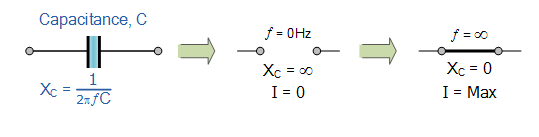# 电容电抗

RC 网络教程中，我们看到当直流电压施加到电容器时，电容器本身从电源吸取充电电流并充电至等于所施加电压的值。

### 电容电抗

![电容电抗](/img/filter/capacitive reactance.png)

• Xc = 以欧姆表示的电容电抗（Ω）
• π = 3.142
• ƒ =以赫兹为单位的频率（Hz）
• C = 以法拉为单位的电容（F）

## 电容电抗实例 No1

• 频率为 1kHz：

$$X c = \frac { 1 } { 2 \pi f C } = \frac { 1 } { 2 \pi \times 1000 \times 220 \times 10 ^ { - 9 } } = 723.4 \Omega$$

• 频率为 20kHz：

$$X c = \frac { 1 } { 2 \pi f C } = \frac { 1 } { 2 \pi \times 20000 \times 220 \times 10 ^ { - 9 } } = 36.2 \Omega$$

### 电容电抗 vs 频率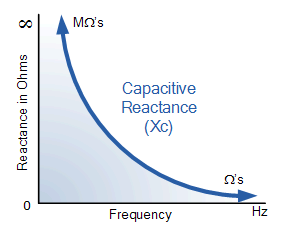## 电容电抗实例 No2

2.2uF 电容在哪个频率下的电抗值为 200Ω？

$$f = \frac { 1 } { 2 \pi C X c } = \frac { 1 } { 2 \pi \times 2.2 \times 10 ^ { - 6 } \times 200 } = 361.7 H z$$

## 电容电抗实例 No3

$$C = \frac { 1 } { 2 \pi f X c } = \frac { 1 } { 2 \pi x 50 \times 200 } = 15.92 u F$$

## 分压器修订版

### 分压网络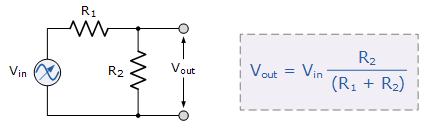### 低通滤波器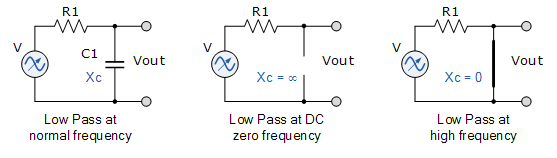### 高通滤波器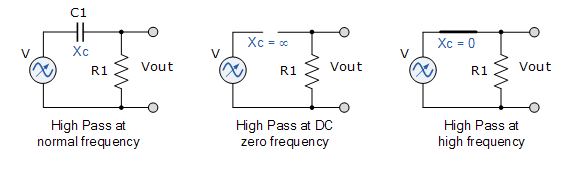## 电容电抗总结